# Seminar 7

In this seminar, we will use Y.RData and VaR.RData files that we created in the Seminars 2 and 7. We will discuss how to implement backtesting. Please review the methods of backtesting in the lecture.

The plan for this week:

The following sections and subsections are materials for students to learn by themselves: Multivariate EWMA and HS VaR and Stress Testing.

We first load the packages for this seminar:

In :
library(lubridate)
library(rugarch)


We will continue building VaR forecasts for the returns of Microsoft. You should load the VaR data frame from the previous seminar, which should contain the columns:

• HS300
• HS1000
• HS2000
• EWMA_VaR
In :
# Load files from the previous seminar

y <- Y$MSFT dates <- Y$date

# Parameters
portfolio_value = 1000
p = 0.05 #probability for VaR


## GARCH VaR¶

To implement VaR forecast using GARCH for a given estimation window, we need to recursively fit the specified GARCH model to the moving estimation window to get the optimal parameters, and use these to forecast conditional volatility for the following day. Once we have the conditional volatility, we estimate VaR using the inverse cdf for the specified probability and the portfolio value. For example, if the estimation window is 1000 days, we should:

1. Use data from day 1 to day 1000 to fit the GARCH model
2. Extract the optimal coefficients
3. Find $\hat{\sigma}_{t+1}$ using the GARCH equation
4. Compute VaR by: $\textrm{VaR}_{t+1}(p) = -\hat{\sigma}_{t+1} F_{R}^{-1}(p)\ \vartheta$

Note that we will be fitting thousands of GARCH models, so this will take a long time to run.

We want to forecast GARCH VaR using estimations windows of 300 and 2000 days. The best way to do so is to write a function:

In [ ]:
# Steps inside the function:
# Take as argument
# GARCH spec, here the default
# Probability, here 0.05
# Portfolio value, here 1000
# Estimation window, here 1000
spec <- ugarchspec()
p <- 0.05
portfolio_value <- 1000
WE <- 1000

# Determine number of observations
n <- length(y)

# Initialize empty VaR vector

VaR <- rep(NA, n)

# Do a loop for the forecast
for (i in 1:(n-WE)){

# Subset the dataset to the estimation window
window <- y[i:(i+WE-1)]

# Fit the GARCH
res <- ugarchfit(spec = spec, data = window, solver = "hybrid")

# Save coefficients
omega <- coef(res)['omega']
alpha <- coef(res)['alpha1']
beta <- coef(res)['beta1']

# Estimate sigma2 using the last observation of window
sigma2 <- omega + alpha*tail(window,1)^2 + beta*tail(res@fit$var,1) # Allocate the VaR forecast in the vector VaR[i+WE] <- -sqrt(sigma2) * qnorm(probability) * portfolio_value }  In : # Function that creates a GARCH forecast DoGARCH <- function(y, spec, probability = 0.05, portfolio_value = 1, WE = 1000){ # GARCH function that takes as argument: # y: A vector of returns, ordered by date # spec: The ugarchspec object with the GARCH specification # probability: The probability to be used for VaR - Default 5% # portfolio_value: The portfolio value - Default 1 # WE: Estimation window for the forecast - Default 1000 days # To calculate elapsed time, first get the current time old <- Sys.time() # Print message cat("Doing GARCH VaR forecast", "\n", "Estimation window:", WE, "\n", "Number of observations:", length(y), "\n", "VaR probability:", probability, "\n", "Portfolio value:", portfolio_value) # Number of observations n <- length(y) # Initialize empty VaR vector VaR <- rep(NA, n) # Do a loop for the forecast for (i in 1:(n-WE)){ # Subset the dataset to the estimation window window <- y[i:(i+WE-1)] # Fit the GARCH res <- ugarchfit(spec = spec, data = window, solver = "hybrid") # Save coefficients omega <- coef(res)['omega'] alpha <- coef(res)['alpha1'] beta <- coef(res)['beta1'] # Estimate sigma2 using the last observation of window sigma2 <- omega + alpha*tail(window,1)^2 + beta*tail(res@fit$var,1)

# Allocate the VaR forecast in the vector
VaR[i+WE] <- -sqrt(sigma2) * qnorm(probability) * portfolio_value
}

# Get the new time and print the elapsed time
time <- difftime(Sys.time(), old, units = "secs")
cat("\n", "Elapsed time:", round(time,4), "seconds")

# Return the VaR vector
return(VaR)
}


Doing the GARCH VaR forecast for estimation windows of 300 and 2000 days:

In :
# Create specification
spec <- ugarchspec(
variance.model = list(garchOrder= c(1,1)),
mean.model = list(armaOrder = c(0,0), include.mean=FALSE)
)

In :
# GARCH VaR for 300 days
GARCH300 <- DoGARCH(y, spec = spec, probability = 0.05, portfolio_value = 1000, WE = 300)

Doing GARCH VaR forecast
Estimation window: 300
Number of observations: 8126
VaR probability: 0.05
Portfolio value: 1000
Elapsed time: 435.2304 seconds

Since the code takes a long time to run, we can save the output to avoid having to run it again:

In :
# Saving the output
save(GARCH300, file = "GARCH300.RData")

In :
# GARCH VaR for 2000 days
GARCH2000 <- DoGARCH(y, spec = spec, probability = 0.05, portfolio_value = 1000, WE = 2000)

Doing GARCH VaR forecast
Estimation window: 2000
Number of observations: 8126
VaR probability: 0.05
Portfolio value: 1000
Elapsed time: 746.078 seconds
In :
# Saving the output
save(GARCH2000, file = "GARCH2000.RData")

In :
# If we have it already saved, we can load it by:


We can plot them together:

In :
# Bind into a matrix
GARCH_VaR <- cbind(GARCH300, GARCH2000)

# Plot and modify axis to include dates
matplot(dates, GARCH_VaR, type = "l", lty = 1, col = 1:2, xaxt = "n", main = "GARCH VaR", xlab = "Date", ylab = "VaR USD")
axis.Date(1, at = seq(min(dates), max(dates), by = "years"))

# Legend
legend("topright", legend = c("WE: 300", "WE: 2000"), lty = 1, col = 1:2)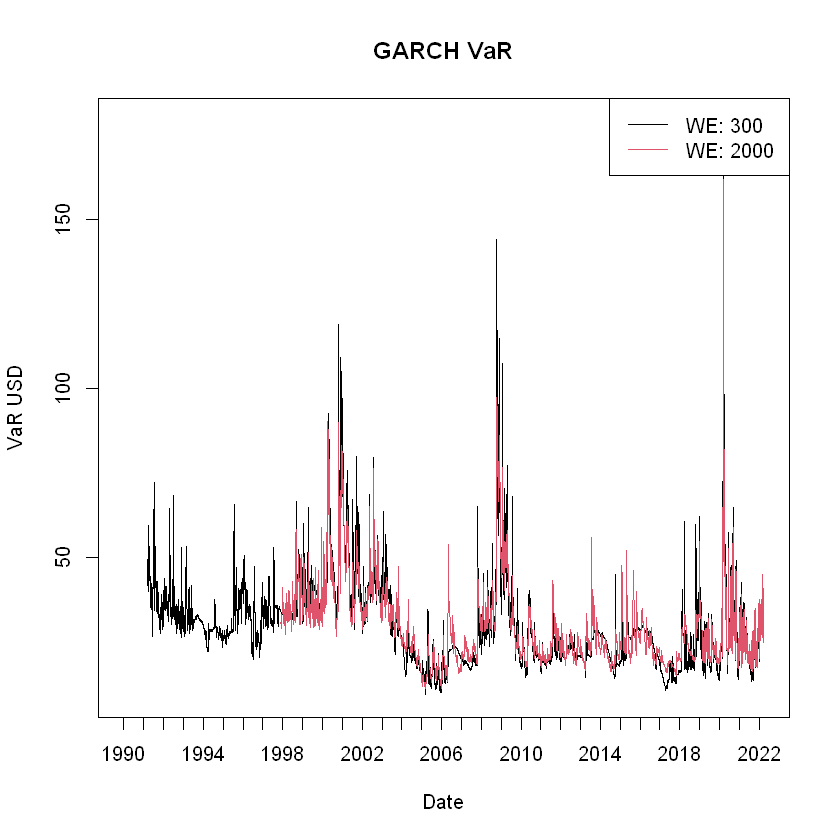## Analyze and compare VaR forecasts¶

We now have 6 different VaR forecasts:

1. EWMA using WE = 30 days
2. HS using WE = 300 days
3. HS using WE = 1000 days
4. HS using WE = 2000 days
5. GARCH using WE = 300 days
6. GARCH using WE = 2000 days

In order to analyze and compare them, let's put them together in a matrix:

In :
# Combining all VaR forecasts
# VaR is load from .RData file includes VaR for HS300, HS1000, HS2000 and EWMA
VaR <- cbind(VaR, GARCH300, GARCH2000)


First, let's compare the means and standard deviation for each forecast. To get the standard deviation of each column, we can use the apply(), which takes as input a matrix, a function to be applied to each row/column, and argument MARGIN: 1 for rows and 2 for columns.

Note that our models have different estimation windows, so the comparison is not completely fair because the number of NA is inconsistent accross the columns. We need to use the option na.rm = TRUE, all NA will be removed before computation:

In :
# Means for each forecast
round(colMeans(VaR, na.rm = TRUE),3)

# Standard deviations - We
round(apply(VaR, MARGIN = 2, sd, na.rm = TRUE))

HS300
29.13
HS1000
28.726
HS2000
28.84
EWMA_VaR
30.048
GARCH300
30.204
GARCH2000
29.608
HS300
11
HS1000
7
HS2000
5
EWMA_VaR
13
GARCH300
13
GARCH2000
12

First, we see the mean is quite similar for all models. However, the standard deviations are quite different. We see that for the Historical Simulation models, a larger estimation window implies a smaller standard deviation of the forecasts. For the GARCH model, the effect of the size of the estimation window on the standard deviation of forecasts is not clear.

Let's use matplot() to plot the six forecasts:

In :
# Plot all
matplot(dates, VaR, type = "l", lty = 1, col = 1:6, xaxt = "n", main = "VaR forecasts", xlab = "Date", ylab = "VaR USD")
axis.Date(1, at = seq(min(dates), max(dates), by = "years"))

# Legend
legend("topright", legend = colnames(VaR), lty = 1, col = 1:6)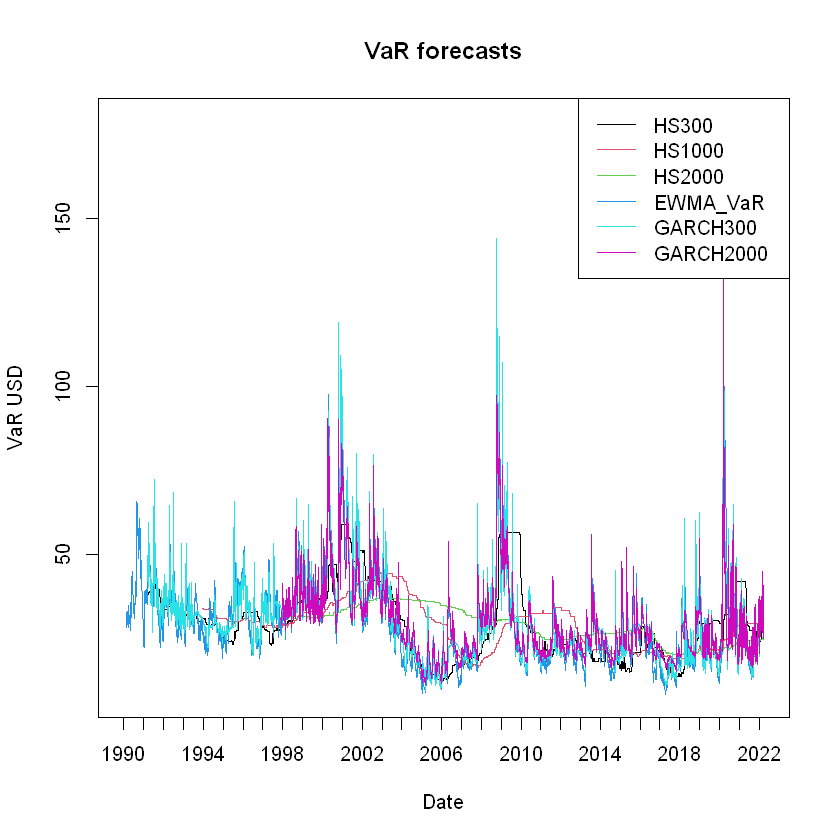Since all our models have different estimation windows, we focus on the most restrictive one.

We use the is.na() function. The function will return a logical vector of same length as the input. TRUE for this elements marked NA.

In :
# Find maximum estimation window
windows <- colSums(is.na(VaR))

#restrict to largest estimation window
start <- max(windows) + 1
end <- length(dates)

# Plot all
matplot(dates[start:end], VaR[start:end,], type = "l", lty = 1, col = 1:6, xaxt = "n",
main = "VaR forecasts", xlab = "Date", ylab = "VaR USD")
axis.Date(1, at = seq(dates[max(windows)], max(dates), by = "years"))

# Legend
legend("topright", legend = colnames(VaR), lty = 1, col = 1:6)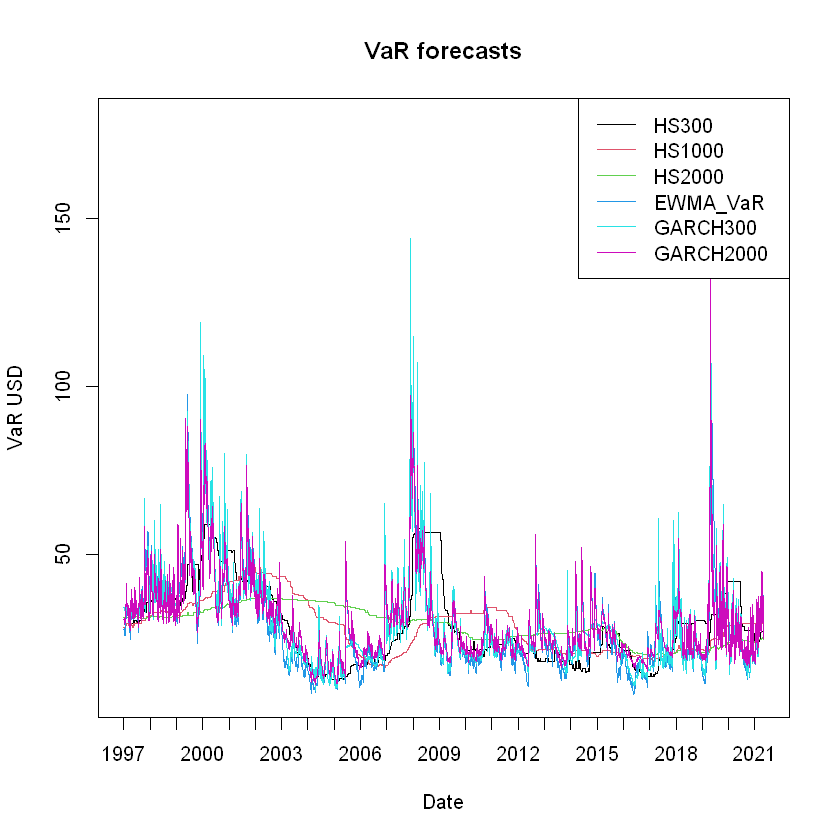## Backtesting¶

### Violation Rations¶

In order the forecasts of our different models, we will perform a backtest. This involves comparing the forecasted VaR for time $t$ to the realized return for that same date. If the return in time $t$ is a loss larger than the forecasted VaR, we count this as a violation:

VaR violation: an event such that $$\eta _{t} = \begin{cases} 1 & \text{if y_t ≤ -VaR_t} \\ 0 & \text{if y_t > -VaR_t}\\ \end{cases}$$

We proceed to count the violations in the Testing Window $W_T$, which is the length of the dataset minus the Estimation Window:

Violations: $v_1$
Non-violations: $v_0$

Where:

$$v_1 = \sum_{t=1}^{W_T} \eta_t$$$$v_0 = W_T - v_1$$

The main tool we use for backtesting are the violation rations. We compare the observed number of VaR violations with the expected number. If we are calculating a 5% VaR, we expect to see $5\% \times W_T$ violations.

We define the Violation Ratio as:

$$\text{VR} = \frac{\text{Observed violations}}{\text{Expected violations}} = \frac{v_1}{p\times W_T}$$

If the violation ratio is greater than one, it means we observe more violations than we expect, so our VaR model is underforecasting risk. On the other hand, if it is smaller than one, we are overforecasting risk.

We expect $\text{VR}=1$, but how can we interpret the $\text{VR}$ from a model? A useful rule of thumb is:

• If $\text{VR} \in [0.8,1.2]$ the model is good
• If $\text{VR} \in [0.5,0.8]\ \text{or}\ \text{VR} \in [1.2,1.5]$ the model is acceptable
• If $\text{VR} \in [0.3,0.5]\ \text{or}\ \text{VR} \in [1.5,2]$ the model is bad
• If $\text{VR} < 0.5\ \text{or}\ \text{VR} > 2$ the model is useless

### Backtesting in R¶

We will now calculate the Violation Ratios for each of our models:

In :
# Backtesting and Violation Ratios

# Let's transform VaR to a data.frame
VaR <- as.data.frame(VaR)

# Initialize a Violations data.frame, same dim and colnames as VaR, fill with NA
Violations <- VaR
Violations[] <- NA

dim(Violations)

1. 8126
2. 6

We are going to populate the Violations matrix using a loop that compares each day's VaR forecast for every model with the realized returns on that day. We will compare each value in VaR with the realized return:

In :
# Populating the Violations matrix

# For every model (columns in VaR) restricted to largest estimation window
for(i in 1:dim(VaR)){

# Fill the column in Violations with TRUE/FALSE
# TRUE if the realized return is lower than VaR
# FALSE otherwise
Violations[,i] <- (y*portfolio_value < -VaR[,i])
}
# Restrict to largest estimation window
Violations[1:(start-1),] <- NA


We can use the which() function to see where the Violations happened in every model:

In :
# Find where violations happened
dates[which(Violations$EWMA_VaR)]  Let's pick one of the days where HS2000 is violated and see if the other models also violate VaR: In : # Get a random day where EWMA VaR is violated using sample() # sample() returns specified size of elements from input random_day <- sample(dates[which(Violations$HS2000)],1)

# Find the index in dates using which()
day_index <- which(dates == random_day)

# See that row in Violations
paste0("Violation for HS2000 on ",random_day)
Violations[day_index,]

'Violation for HS2000 on 2000-03-30'
A data.frame: 1 × 6
HS300HS1000HS2000EWMA_VaRGARCH300GARCH2000
<lgl><lgl><lgl><lgl><lgl><lgl>
2590FALSETRUETRUEFALSEFALSEFALSE

We can easily plot the VaR forecasts and add points where the Violations happened using the points() function.

We can use logical vectors to subset our objects to where violations happened:

In :
# Plotting the violations
plot(dates[start:end], VaR$EWMA_VaR[start:end], type = "l", main = "EWMA VaR with violations",) # Add points where the violations happened points(dates[Violations$EWMA_VaR], VaR$EWMA_VaR[Violations$EWMA_VaR], pch = 16, col = "red")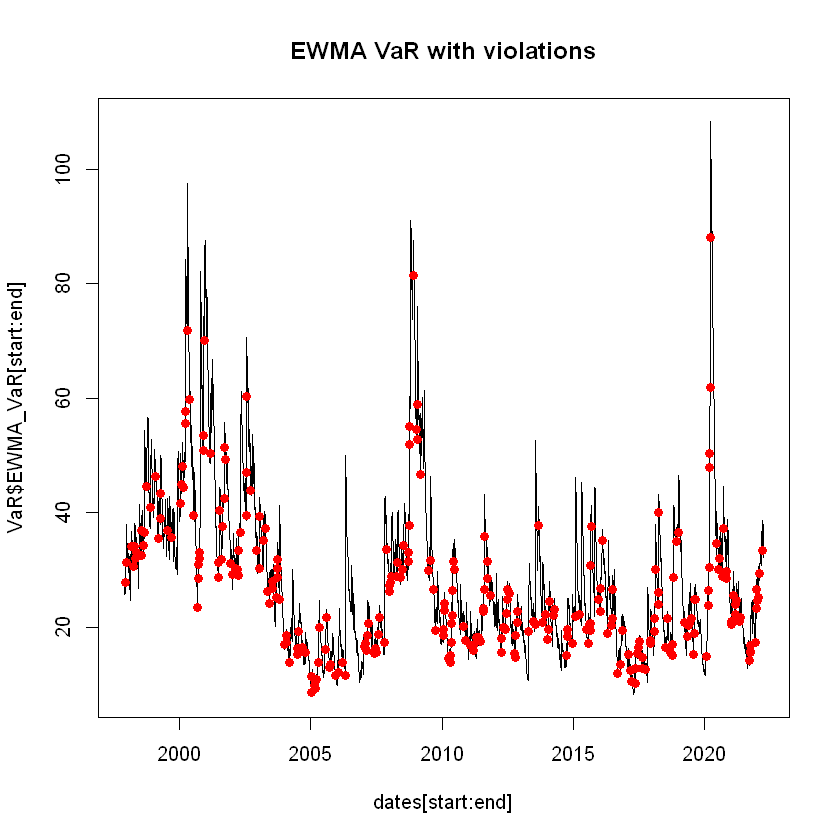Using the & operator, let's see if they are dates for which all our models have a VaR violation. We can use apply() and the all() function, which checks if all elements are TRUE:

In :
# Check dates where all models have a violation
# restrict to largest estimation window
w <- apply(Violations, 1, all)


We can use sum() to cound the number of days where all models have a violation:

In :
# Days where all models have a violation
# na.rm =TRUE means we will firstly remove all NA elements
sum(w, na.rm = TRUE)

139

Let's plot these days with the returns:

In :
# Plotting the returns and adding the days where all models had a violation
plot(dates, y, main = "Microsoft returns", type = "l", lwd = 2, las = 1,
xlab = "Date", ylab = "Returns")
points(dates[w], y[w], pch = 16, col = "red")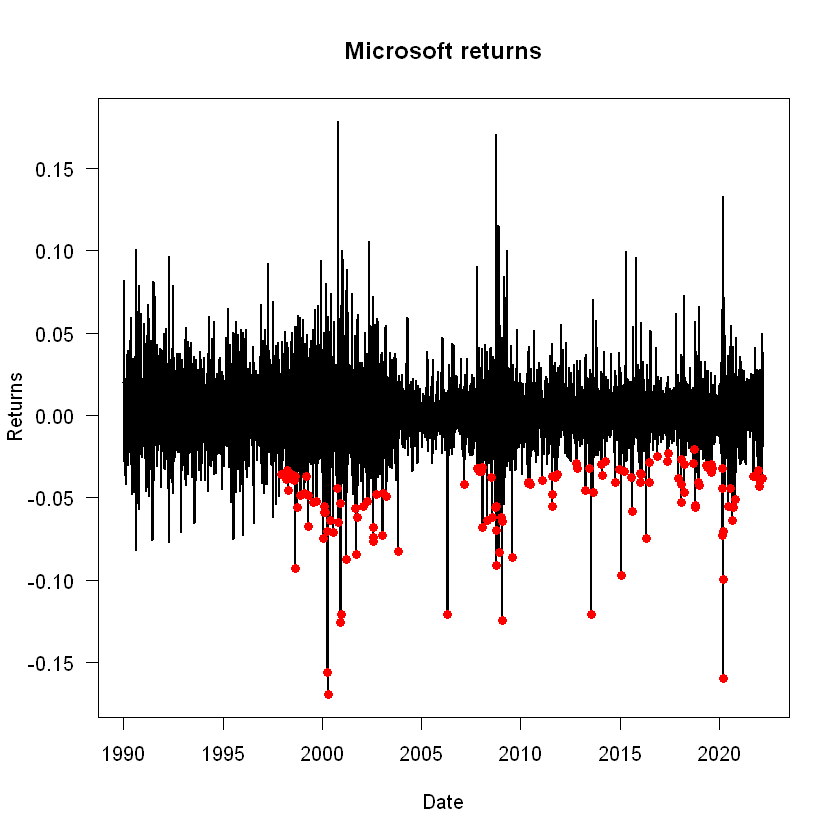To compare the models to each other, we can count the number of violations for every model using colSums():

In :
# Counting Violations by model
colSums(Violations, na.rm = TRUE)

HS300
327
HS1000
338
HS2000
317
EWMA_VaR
280
GARCH300
267
GARCH2000
245

Now we can create a VR object that holds the Violation Ratio for every model:

In :
# Creating a Violation Ratio object

# Remove the rows with NA
Violations <- Violations[!is.na(Violations[,1]),]

# Get the column sums
V <- colSums(Violations)

# Calculate expected violations
EV <- dim(Violations)*p

# Violation Ratios
VR <- V/EV

# Call object, rounding to 3 decimals
round(VR,3)

HS300
1.068
HS1000
1.103
HS2000
1.035
EWMA_VaR
0.914
GARCH300
0.872
GARCH2000
0.8
In :
# We can write a function that uses our rule of thumb to assess the model
model_assessment <- function(VR) {
if (VR > 0.8 & VR < 1.2) {
paste0(names(VR), "Model is good")
} else if ((VR > 0.5 & VR <= 0.8) | (VR > 1.2 & VR <= 1.5)) {
paste0(names(VR), "Model is acceptable")
} else if ((VR > 0.3 & VR <= 0.5) | (VR > 1.5 & VR <= 2)) {
} else {
paste0(names(VR), "Model is useless")
}
}

In :
# We can use sapply(), the vector version of apply()
sapply(VR, model_assessment)

HS300
'Model is good'
HS1000
'Model is good'
HS2000
'Model is good'
EWMA_VaR
'Model is good'
GARCH300
'Model is good'
GARCH2000
'Model is acceptable'

All our models fall under the good category except for the GARCH with 2000 days as estimation window, which is only acceptable. The best performing model under this criteria is:

In :
# Best performing - VR closest to 1
sort(round(abs(VR-1),3))

HS2000
0.035
HS300
0.068
EWMA_VaR
0.086
HS1000
0.103
GARCH300
0.128
GARCH2000
0.2

The model with the best Violation Ratio is the Historical Simulation with 2000 days for Estimation Window. This is interesting since it was the model with the smallest standard deviation.

## Multivariate EWMA and HS VaR (Not covered in class)¶

We will now perform a Multivariate VaR forecast using EWMA and HS for Microsoft, JP Morgan, and Intel, using an estimation window of $W_E = 1000$:

In :
# Multivariate EWMA and HS VaR

# Determine a vector of portfolio weights
w <- c(0.5, 0.2, 0.3)

# EWMA VaR
multi_y <- Y[,c("MSFT", "JPM", "INTC")]
multi_y <- as.matrix(multi_y)
n <- dim(multi_y)
K <- dim(multi_y)

# Number of variables
nvar <- K+K*(K-1)/2
EWMA <- matrix(NA, nrow = n, ncol = nvar)
lambda <- 0.94
S <-- cov(multi_y)
# Fill initial row, order:
# 1st col: MSFT Variance, 2nd: JPM Variance, 3rd: INTC Variance
# 4th: MSFT-JPM, 5th: MSFT-INTC, 6th: JPM-INTC

EWMA[1,] <- c(S[1,1], S[2,2], S[3,3], S[1,2], S[1,3], S[2,3])
colnames(EWMA) <- c("MSFT", "JPM", "INTC", "MSFT-JPM", "MSFT-INTC", "JPM-INTC")

A matrix: 6 × 6 of type dbl
MSFTJPMINTCMSFT-JPMMSFT-INTCJPM-INTC
-0.0004000083-0.0005555709-0.0005736652-0.0001897728-0.0002723314-0.0002236988
NA NA NA NA NA NA
NA NA NA NA NA NA
NA NA NA NA NA NA
NA NA NA NA NA NA
NA NA NA NA NA NA
In :
# Populating EWMA matrix and creating the portfolio variance

portfolio_var <- rep(NA,n)

for (i in 2:n) {
S <- lambda * S + (1-lambda) * multi_y[i-1,] %*% t(multi_y[i-1,])
EWMA[i,] <- c(S[1,1], S[2,2], S[3,3], S[1,2], S[1,3], S[2,3])
portfolio_var[i] <- t(w) %*% S %*% w
}

# Implement the VaR forecast
# Plot estimation for conditional volatility
EWMA_cond_volatility <- sqrt(portfolio_var)
EWMA_VaR <- -qnorm(p) * EWMA_cond_volatility * portfolio_value

# Burn the first 1000 observations
EWMA_VaR[1:1000] <- NA

In :
# Multivariate HS

# Initialize the vector
HS_VaR <- rep(NA, length = n)

# Do the HS
window <- 1000
for (i in 1:(n-window)) {
# Multiply the matrix y (dimension T x 3) by vector w (dimension 3x1)
# Output is portfolio returns (dimension Tx1)
yp <- multi_y[i:(i+window),] %*% w

# Sort the vector
ys <- sort(yp)

# Position of the 5% quantile
quant <- ceiling(p*length(ys))

# Get VaR
HS_VaR[i+window] <- -ys[quant]*portfolio_value
}


Now that we have implemented both EWMA_VaR and HS_VaR, we can plot them together, find the mean and SD, and evaluate the Violation Ratios:

In :
multi_VaR <- cbind(EWMA_VaR, HS_VaR)

matplot(dates, multi_VaR, type = "l", lty = 1, col = 1:2, xaxt = "n", main = "VaR forecasts", xlab = "Date", ylab = "VaR USD")
axis.Date(1, at = seq(min(dates), max(dates), by = "years"))

# Legend
legend("topright", legend = colnames(multi_VaR), lty = 1, col = 1:2)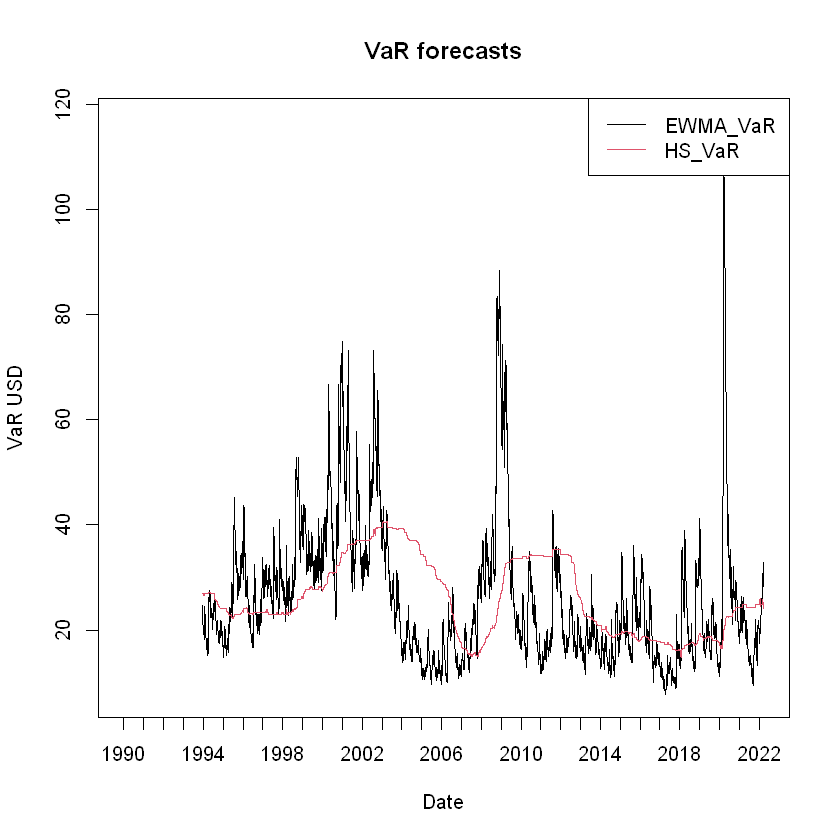In :
# Mean and SD
round(colMeans(multi_VaR, na.rm = TRUE))
round(apply(multi_VaR, 2, sd, na.rm = TRUE))

EWMA_VaR
26
HS_VaR
26
EWMA_VaR
13
HS_VaR
7

As expected, the means are very close to each other but the HS forecast has a lower standard deviation.

In :
# Violation Ratios

# Transform VaR to data frame
multi_VaR <- as.data.frame(multi_VaR)

multi_Violations <- multi_VaR

multi_Violations[] <- NA

for(i in 1:dim(multi_VaR)){
returns <- multi_y %*% w
multi_Violations[,i] <- returns*portfolio_value < -multi_VaR[,i]
}

multi_Violations <- multi_Violations[!is.na(multi_Violations[,1]),]

multi_V <- colSums(multi_Violations)

multi_EV <- dim(multi_Violations)*p

multi_VR <- multi_V/multi_EV

round(multi_VR,3)

model_assessment(multi_VR)
model_assessment(multi_VR)

EWMA_VaR
1.019
HS_VaR
1.092
'EWMA_VaRModel is good'
'HS_VaRModel is good'

Both models are good, with Violation Ratios close to 1.

## Stress Testing (Not covered in class)¶

We are interested in seeing how our models perform in stressful periods of time. So we will define a new time period that consists of the years of the financial crisis (2008-2012), and evaluate our four univariate models then:

In :
# Stress Testing

# Subset for crisis peridos
crisis <- year(dates) >= 2008 & year(dates) < 2013
y_crisis <- y[crisis]
VaR_crisis <- VaR[crisis,]

Violations_crisis <- VaR_crisis
Violations_crisis[] <- NA

for(i in 1:dim(VaR_crisis)){
Violations_crisis[,i] <- y_crisis*portfolio_value < -VaR_crisis[,i]
}

# Remove the rows with NA
Violations_crisis <- Violations_crisis[!is.na(Violations_crisis[,1]),]

# Get the column sums
V_crisis <- colSums(Violations_crisis)

# Calculate expected violations
EV_crisis <- dim(Violations_crisis)*p

# Violation Ratios
VR_crisis <- V_crisis/EV_crisis

# Call object, rounding to 3 decimals
round(VR_crisis,3)

sapply(VR_crisis, model_assessment)

HS300
1.144
HS1000
1.35
HS2000
1.176
EWMA_VaR
1.048
GARCH300
1.064
GARCH2000
0.937
HS300
'Model is good'
HS1000
'Model is acceptable'
HS2000
'Model is good'
EWMA_VaR
'Model is good'
GARCH300
'Model is good'
GARCH2000
'Model is good'

In this test, all models are good except for HS1000. The model with the Violation Ratio closest to 1 is the EWMA, followed by GARCH2000 which was the worst perfoming model when we considered the entire time period.

In :
# Best performing - VR closest to 1
sort(round(abs(VR_crisis-1),3))

EWMA_VaR
0.048
GARCH2000
0.063
GARCH300
0.064
HS300
0.144
HS2000
0.176
HS1000
0.35

## Recap¶

In this seminar we have covered:

• VaR implementation with EWMA, HS and GARCH
• Comparison of different models and estimation windows
• Backtesting using Violation Ratios
• Assessing models based on their VR
• Multivariate VaR forecast and assessment using EWMA and HS
• Stress-Testing

Some new functions used:

• apply()
• sapply()

For more discussion on the material covered in this seminar, refer to Chapter 5: Implementing risk forecasts and Chapter 8: Backtesting and stress testing on Financial Risk Forecasting by Jon Danielsson.

Acknowledgements: Thanks to Alvaro Aguirre and Yuyang Lin for creating these notebooks